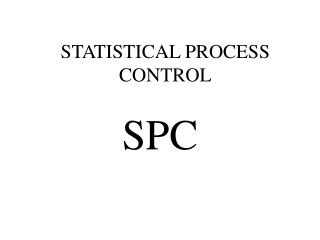Download PresentationSTATISTICAL PROCESS CONTROLSTATISTICAL PROCESS CONTROL - PowerPoint PPT Presentation

Download PresentationSTATISTICAL PROCESS CONTROL
An Image/Link below is provided (as is) to download presentation

Download Policy: Content on the Website is provided to you AS IS for your information and personal use and may not be sold / licensed / shared on other websites without getting consent from its author. While downloading, if for some reason you are not able to download a presentation, the publisher may have deleted the file from their server.

- - - - - - - - - - - - - - - - - - - - - - - - - - - E N D - - - - - - - - - - - - - - - - - - - - - - - - - - -
Presentation Transcript

1. PROCESS IN A STATE OF STATISTICAL CONTROL

2. PROCESS “NOT” IN A STATE OF STATISTICAL CONTROL

3. PROCEDURE TO DETERMINE IF PROCESS IS IN A STATE OF STATISTICAL CONTROL • 1. Randomly sample “n” units throughout the day (can be every 5 minutes, every 30 minutes, etc. - whatever is appropriate for your case) • 2. For each sample, calculate “X-BAR” to estimate the mean and “R (range)” to estimate variability. NOTE: THE RANGE CAN BE USED TO ESTIMATE THE STANDARD DEVIATION.

4. PROCEDURE TO DETERMINE IF PROCESS IS IN A STATE OF STATISTICAL CONTROL • 3. After collecting the 20-25 samples, calculate X-BAR-BAR (the average of all the X-BARs) and R-BAR (the average of all the ranges) • 4. Using X-BAR-BAR and R-BAR, calculate the control limits for your X-BAR and R Control Charts. • X-BAR CONTROL LIMITS: (X-BAR-BAR) + A2 (R-BAR) • R CONTROL LIMITS: D3 (R-BAR) and D4 (R-BAR)

5. CONTROL CHART CONSTANTS

6. PROCEDURE TO DETERMINE IF PROCESS IS IN A STATE OF STATISTICAL CONTROL • 5. For the R Control Chart, plot all R values on the R Control Chart to see if all the Ranges are “between the control limits”. If they are, then your process is considered to be in a state of statistical control (as far as the variability of the process is concerned) • 6. For the X-BAR Control Chart, plot all the X-BARs on the X-BAR control chart to see if all the X-BARs are “between the control limits”. If they are, then your process is considered in a state of statistical control (as far as the average of the process is concerned) • NOTE 1: If the R chart is not stable, do not try to evaluate stability of the X-BAR chart. (always look at R chart first) • NOTE 2: There are other rules available to help determine statistical stability, other than all observations being within the control limits. These are normally referred to as the WECO rules.

7. PLOT DATA ON CONTROL CHARTS CALCULATED TO DETERMINE STATISTICAL STABILTIY (EXAMPLE: PROCESS IN CONTROL)

8. PLOT DATA ON CONTROL CHARTS CALCULATED TO DETERMINE STATISTICAL STABILTIY (EXAMPLE: PROCESS NOT IN CONTROL)

9. PROCEDURE TO DETERMINE IF PROCESS IS IN A STATE OF STATISTICAL CONTROL • 7. If process is determined to be “NOT STABLE”, then stop and find out what an assignable cause might be, fix, and then repeat the complete process of collecting new data. DO NOT USE THESE ORIGINAL CONTROL CHARTS THAT REFLECTED AN UNSTABLE PROCESS TO MONITOR FUTURE PRODUCTION. • 8. If the process is determined to be “STABLE”, then you may use the control charts you developed to monitor future production to insure that the process REMAINS stable.

10. IF PROCESS IS STABLE, EXTENT CONTROL CHARTS TO MONITOR “FUTURE” DATA • 9. AT ANY FUTURE POINT IN TIME, IF ANY X-BAR OR R VALUE IS NOT BETWEEN CONTROL LIMITS, OR VIOLATES ONE OF THE WECO RULES, THEN STOP THE PROCESS AND FIND OUT WHAT WENT WRONG!